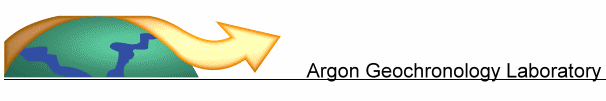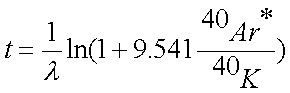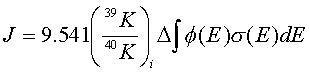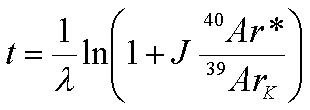40Ar/39Ar Dating Method Since 40Ar/39Ar technique of K-Ar dating was first established in 1960s,  the technique has been gradually developed and utilized with marked success to investigate a wide range of geological problems.  In particular, the knowledge obtained from 40Ar/39Ar dating measurements has provided a powerful approach to the study of kinetic processes in geological terranes and thermal histories of terrestrial and extraterrestrial samples. 　 The 40Ar/39Ar dating method is a variation of the conventional K-Ar method which is based on the decay scheme of 40K to 40Ar with a half-life of 1.3 billion years. It requires bombardment of samples with fast neutrons in a nuclear reactor to convert 39K (the most abundant potassium isotope) to 39Ar. Samples are then fused in an ultra-high vacuum gas extraction system and 39Ar/40Ar ratio determined by a gas-source mass-spectrometer. Calibration of the reactor flux with standards of known age allows the amount of 39K (and consequently the 40K) in the sample to be determined by analyzing 39Ar. Natural decay of 40K generates radiogenic 40Ar (40Ar*) with time.     With utilizing the measurements of 40K and 40Ar cumulated in the sample,  40Ar/39Ar  date (t) can be estimated with using the following equation:where l = 5.543 x 10-10 a-1                                          (1) The above equation stands as the central for K-Ar dating, so-called age equation. In 40Ar/39Ar dating, the sample has to be irradiated in reactor and 39K in sample can be converted to 39Ar.  Through the analysis of 39Ar and adequate interference corrections, the amount of 40K in the sample can be calculated via the following equation:(2) where, 39Ark: amount of 39Ar generated from irradiation of 39K in sample in reactor, D: irradiation duration, f(E): neutron flux at energy E,  s(E): neutron capture cross section at E for the 39K(n,p)39Ar reaction, and ( 40K/39K)i: isotopic abundance ratio of K. Combing Eqns. (1) and (2), Eqn. (1) becomes:(3) By assigning, Eqn. (3) becomes(4) Practically, J value can be obtained with analyzing a sample of accurately known K/Ar age (standard mineral or irradiation monitor), be irradiated together with the unknown sample. In this case, the age of the unknown sample can be calculated from the isotopic ratio  (40Ar*/39ArK) obtained from the analysis.   40Ar/39Ar technique has the following advantages over the conventional K/Ar technique 　 Samples do not have to be splitted for separate K and Ar analyses; the parent/daughter abundance are obtained from the same sample aliquot analyzed. This allows accurate ages determination for minerals with low potassium contents such as pyroxene or plagioclase. Error in 40Ar/39Ar dates are generally less than the usual 2-3% error associated with K-Ar dates. The precision of age is determined by the uncertainty in age of standard used to calibrate the neutron flux (usually 1% or less). The precision of relative dates for samples irradiated in the same sample container is limited by uncertainty in flux determination and analytical precision of the mass spectrometer (0.1 to 0.2%). Sample can be incrementally heated and age determined for each temperature step producing 40Ar/39Ar "age spectra". The shape of these spectra can frequently be used to determine whether the K-Ar system has been disturbed or contains a mixed population of discordant ages. Argon isotope data from incremental heating with carefully controlled temperature and time can be used to determine closure temperature for argon system of that particular mineral. By utilizing laser ablation technique, separate mineral grains or grains in thin section can be analysed. This allows accurate determination of ages of microgram to milligram quantities of minerals sample that is difficult to separate or in-situ analysis is desirable. Incremental heating of individual mineral grains with a laser is more rapid and straightforward compared to conventional furnace extraction systems. Pulsed laser probe allows to detect the age zoning in a single mineral grain.  Thus, age profiling can therefore be desirable. Publications: Lo, C.H. and Lee, C.Y.(1994) 40Ar/39Ar Method of K-Ar Age Determination of Geological Samples Using Tsing-Hua Open-Pool (THOR) Reactor. Jour. Geol. Soc. China 37, 143-164. Lo, C.H., Wang, P.-L., Yang, H.-C., Liou, Y.-S. and Tsou, T.-Y. (2001) The Laser 40Ar/39Ar Dating Microprobe of National Taiwan University. Western Pacific Earth Sciences 1, 143-156.